# A Digital Signature Batch Verification Scheme for ElGamal

Batch Screening is a scheme which is used with ElGamal Signature Scheme to improve the performance of verifying a large number of signed messages. In Batch screening, a batch of messages is taken together and verified all at once other than verifying each of them individually which is the standard method. Following is an implementation of a Batch Screening system for ElGamal Signature scheme implemented in Python. Source Code can be found at GitHub here.

### Signing Messages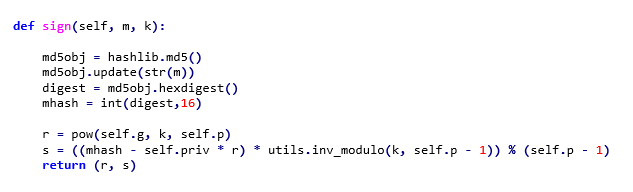A random number k (1<k<p-1 and gcd(k,p-1)=1) and the message to be signed to the function. Hash of the message is calculated using MD5 hash function which is used to create the signature. Signature is created as a tuple (r,s) where,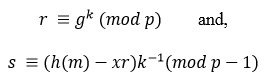In my implementation, I have created 20 such distinct messages and signed each message by calling the sign() function. These messages and signatures are then passed to each function, singleVerify() and batchVerify() for per-message signature verification and batch screening.

### Per-message signature verification (singleVerify() function)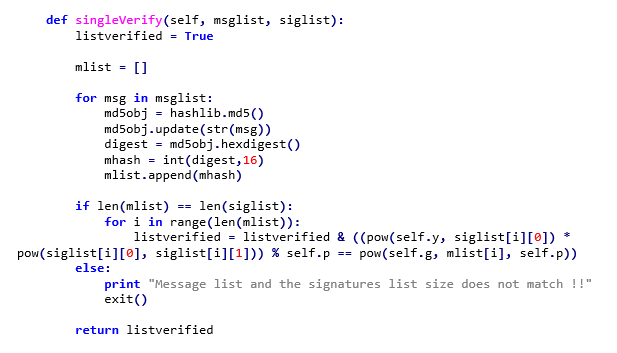In per-message signature verification, each message is verified individually. If all messages are verified successfully, the final result would be returned as True, otherwise False. Verification is done as follows: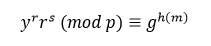### Batch Verification (Screening)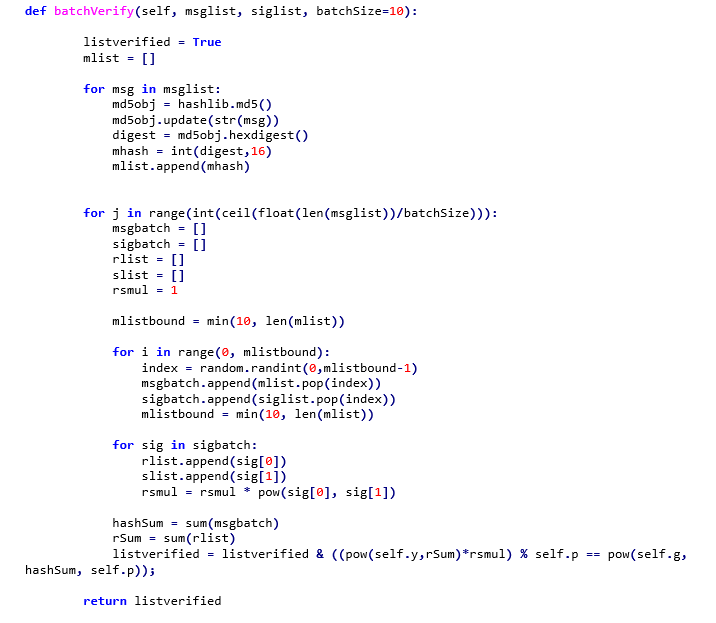In Batch Screening, a batch of messages has been taken at once and verified at once. In this case, I have used the Batch size as 10, hence 10 messages will be verified at once. If all messages are signed properly and integrity not lost, batchVerify() will return true. Even if a single message in the batch is modified, verification will fail for the entire batch of messages. Batch verification is done as follows:

Condition for single message signature verification is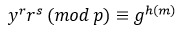For each m_i message in a set of n messages, the condition should be,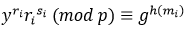For the entire batch of messages,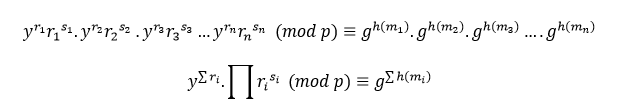An attacker could manipulate adjacent messages so that their changes cancel out in batch verification. This might cause an attack on message integrity getting unnoticed. To prevent this, we need to select a batch of message-signature pairs randomly rather than selecting them sequentially for a single batch.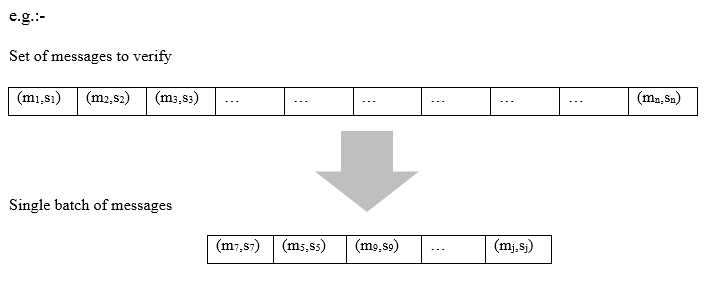I used a random function to select messages randomly as follows:On each batch of messages, validation,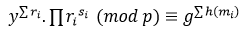is calculated and the final result for the batch will be returned as True if validation successful for each batch.

### Results

Using python time.time() function, I calculate the time it took for each method, Single message signature verification, and batch screening and plotted the results.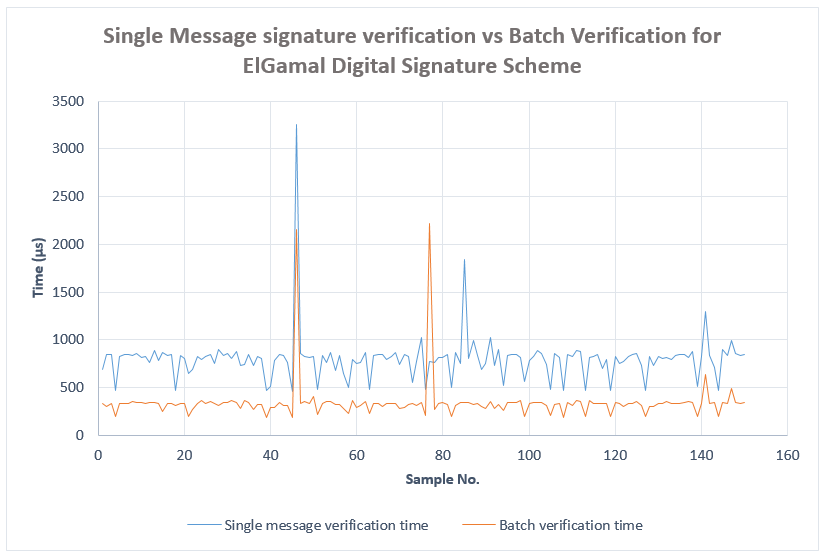According to the graph, we can notice that the time it took to verify all the messages clearly differ in two schemes. This shows that we can gain a significant performance improvement when Batch Screening is used to verify a large number of messages rather than individually verifying messages.

On average, I could notice that Batch screening took 343.224208 µs to verify 20 messages with MD5 as the hashing scheme and with batch size 10. When each message individually verified, it took 800.329844 µs on average for verification. We can clearly see that we have an improvement in verification time by 457.105637 µs on average when Batch screening is used instead of the standard method.

Following graph shows the difference between average verification times for each scheme.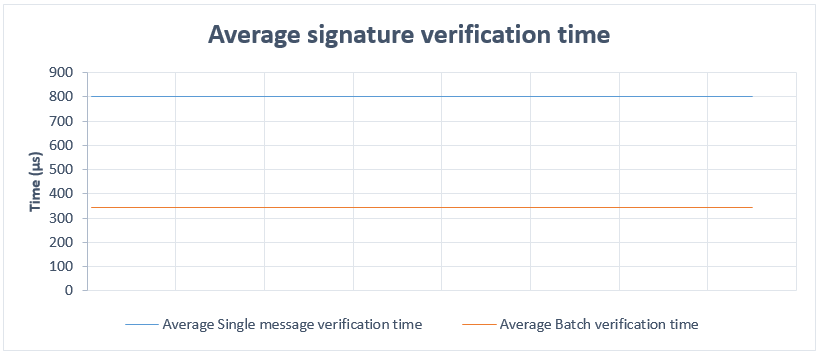Following is the change of Batch Verification time (average) with respect to Batch Size chosen. I changed the batch size by 50 each time and measured the verification time for 1000 messages. It can be noticed that Batch Verification time decreases as the batch size increases. However, the rate of decrease decreases as batch size increases.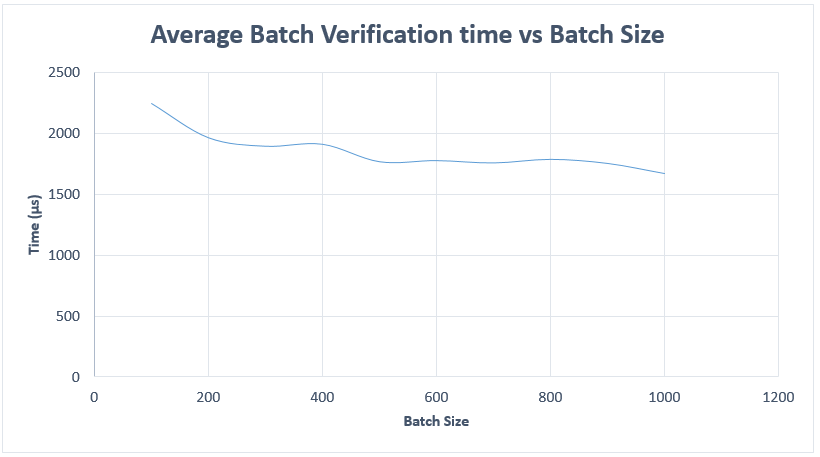Following graph shows how Total time for verification changes as the Total message count increases. We can identify that the increase of verification time is high in Individual message signature verification compared to batch screening. In this experiment, the batch size was kept fixed at 10.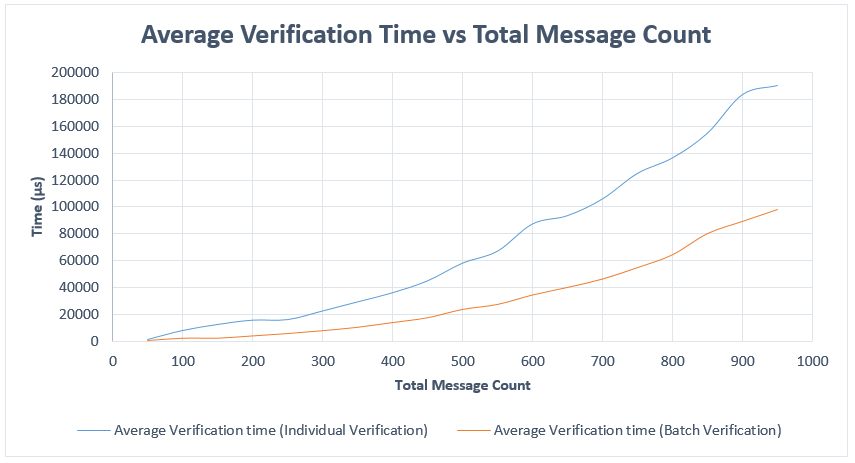By the above results, we can conclude that Batch Screening significantly improves the performance of ElGamal Signature scheme when there are multiple (preferably large number of) messages are to be verified for Digital Signature. As the number of messages increases, the benefit of using Batch screening becomes very larger than that of Individual signature verification.